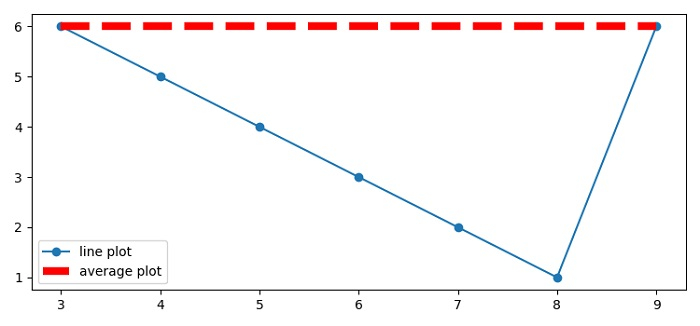# How to draw an average line for a scatter plot in MatPlotLib?

To draw an average line for a plot in matplotlib, we can take the following steps −

• Set the figure size and adjust the padding between and around the subplots.

• Make x and y data points using numpy.

• Use subplots() method to create a figure and a set of subplots.

• Use plot() method for x and y data points.

• Find the average value of the array, x.

• Plot x and y_avg data points using plot() method.

• Place a legend on the figure.

• To display the figure, use show() method.

## Example

import numpy as np
from matplotlib import pyplot as plt

plt.rcParams["figure.figsize"] = [7.50, 3.50]
plt.rcParams["figure.autolayout"] = True

x = np.array([3, 4, 5, 6, 7, 8, 9])
y = np.array([6, 5, 4, 3, 2, 1, 6])

fig, ax = plt.subplots()
ax.plot(x, y, 'o-', label='line plot')
y_avg = [np.mean(x)] * len(x)
ax.plot(x, y_avg, color='red', lw=6, ls='--', label="average plot")

plt.legend(loc=0)
plt.show()

## Output